# Презентация, доклад The adequacy of analysis of linear periodically-time-variable circuits by the frequency symbolic method in the time domain

Вы можете изучить и скачать доклад-презентацию на тему The adequacy of analysis of linear periodically-time-variable circuits by the frequency symbolic method in the time domain. Презентация на заданную тему содержит 13 слайдов. Для просмотра воспользуйтесь проигрывателем, если материал оказался полезным для Вас - поделитесь им с друзьями с помощью социальных кнопок и добавьте наш сайт презентаций в закладки!
Презентации» Физика» The adequacy of analysis of linear periodically-time-variable circuits by the frequency symbolic method in the time domain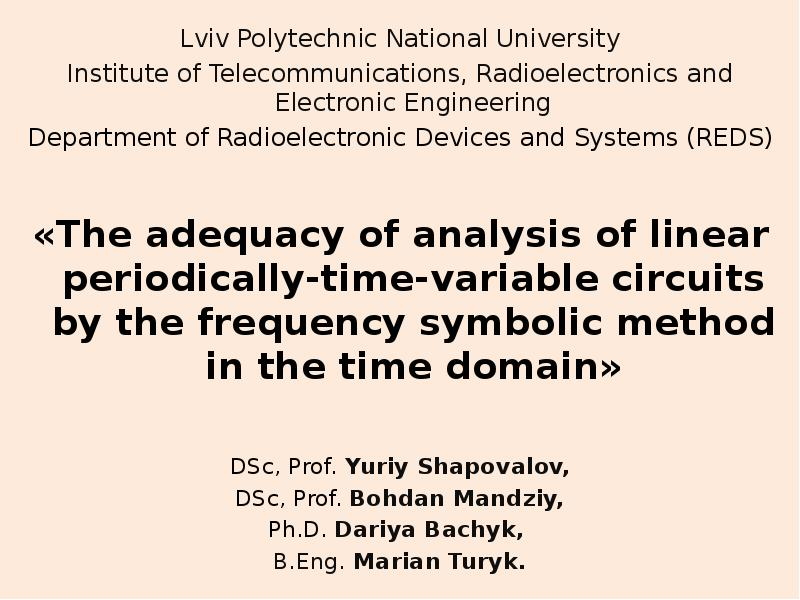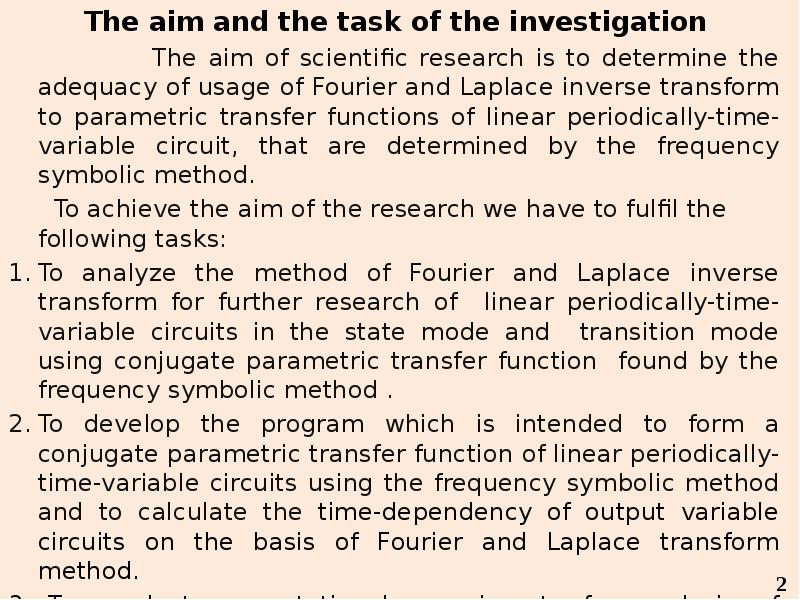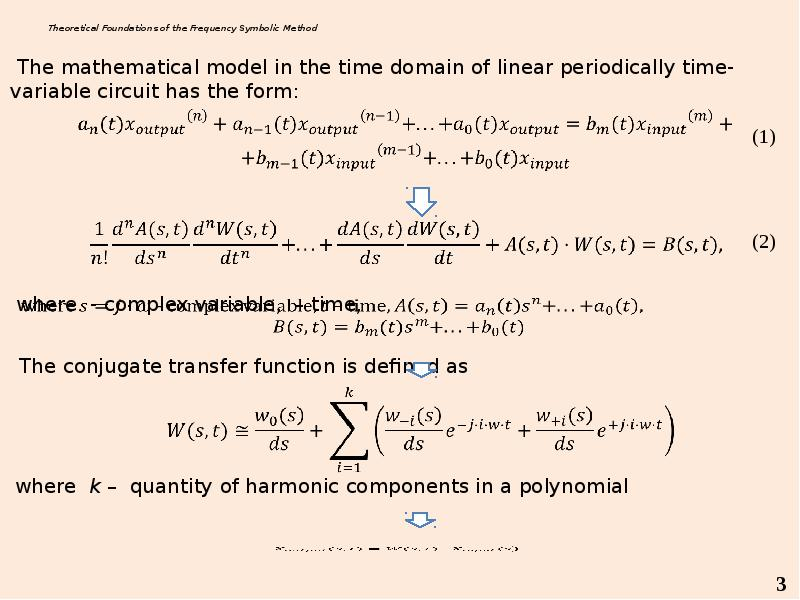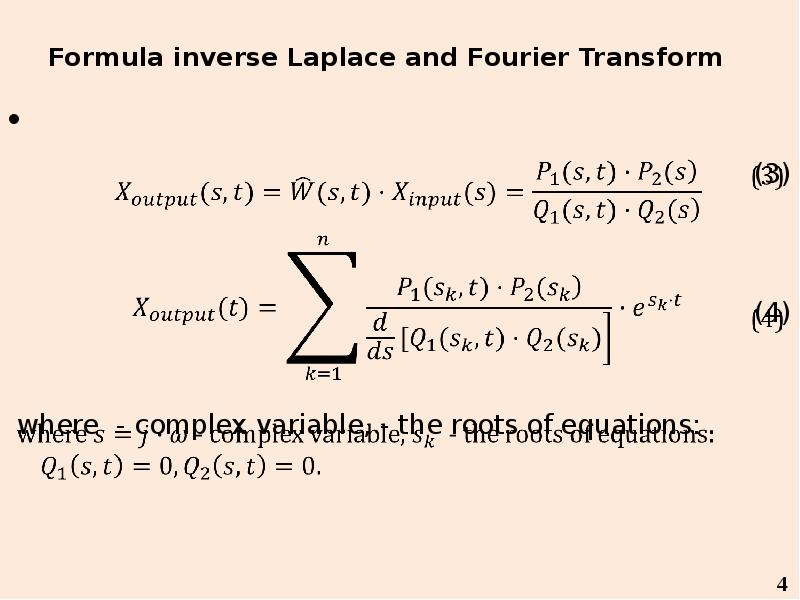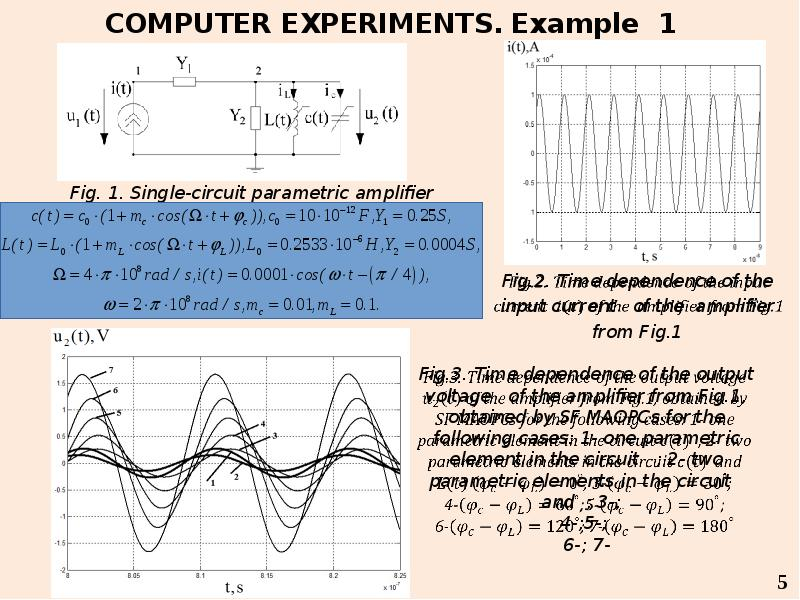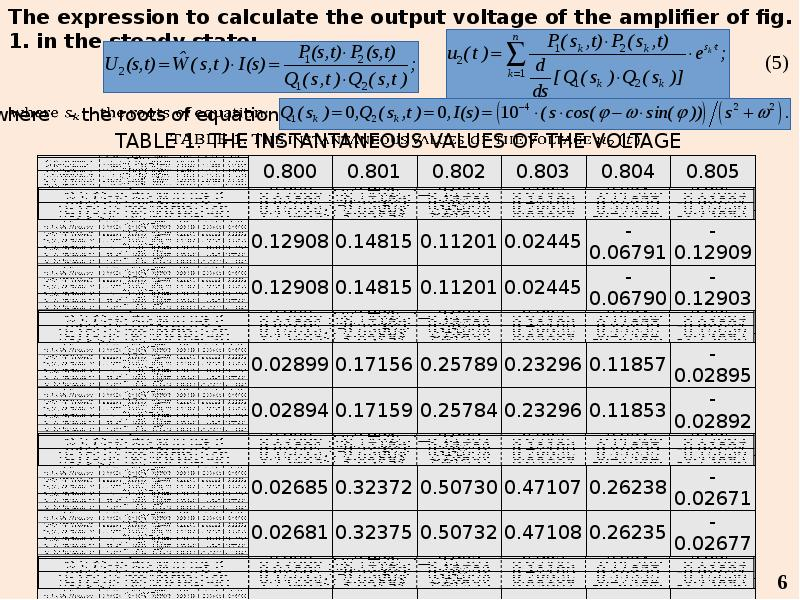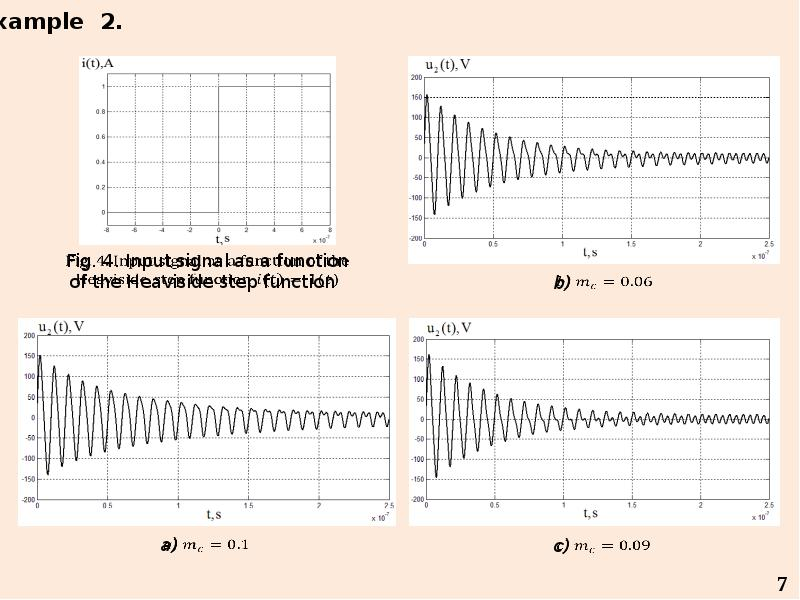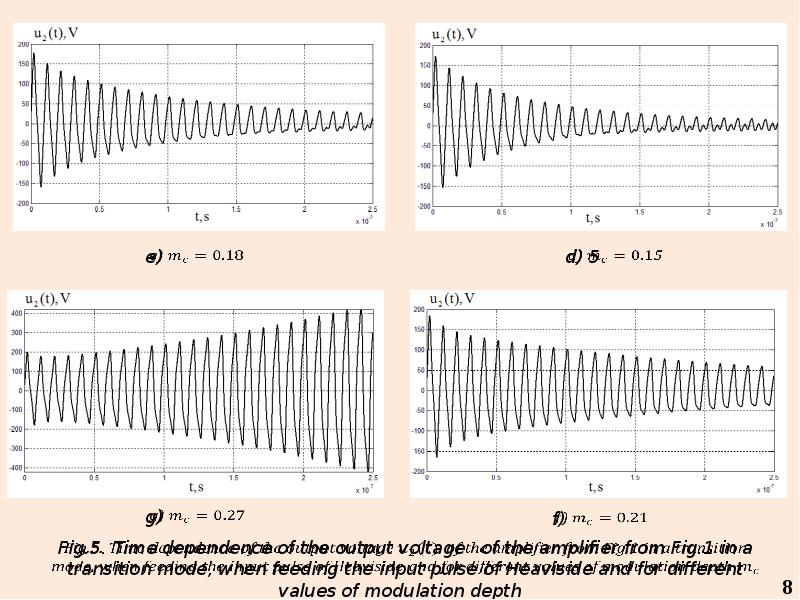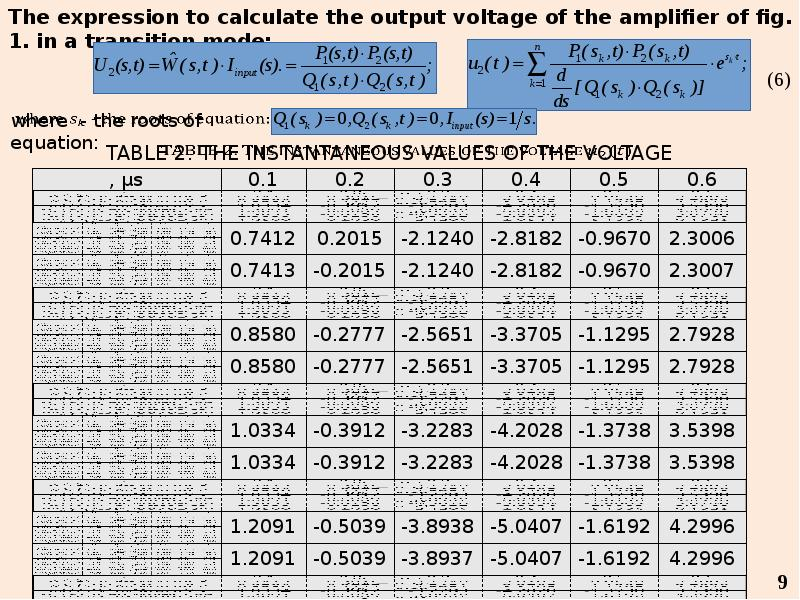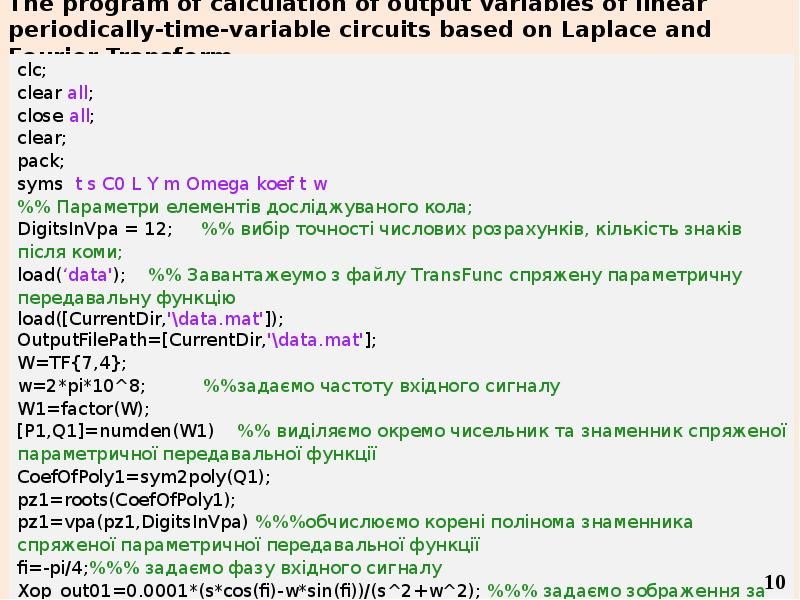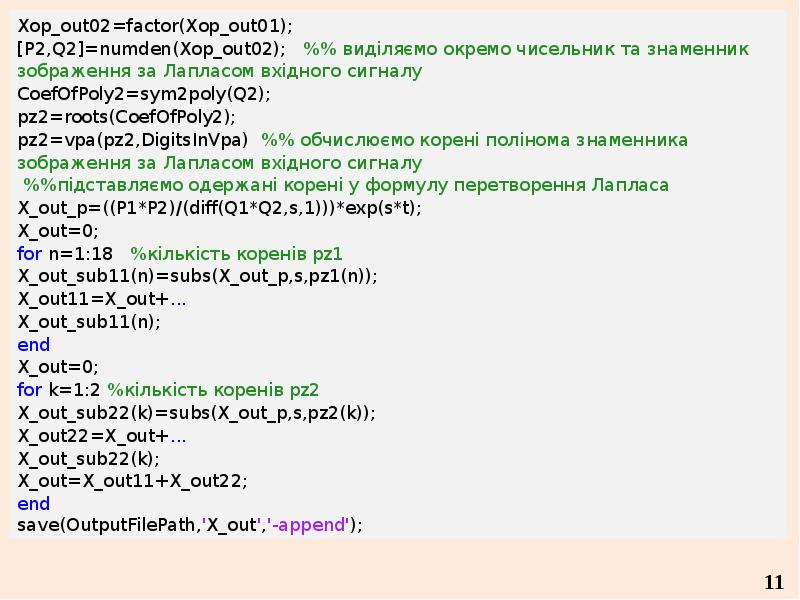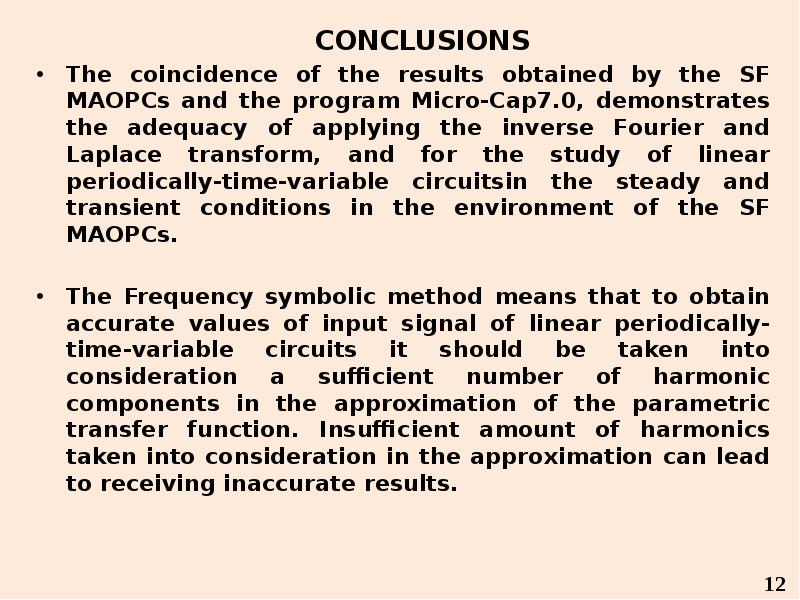Слайды и текст этой презентацииОписание слайда:
Lviv Polytechnic National University Lviv Polytechnic National University Institute of Telecommunications, Radioelectronics and Electronic Engineering Department of Radioelectronic Devices and Systems (REDS) «The adequacy of analysis of linear periodically-time-variable circuits by the frequency symbolic method in the time domain» DSc, Prof. Yuriy Shapovalov, DSc, Prof. Bohdan Mandziy, Ph.D. Dariya Bachyk, B.Eng. Marian Turyk.Описание слайда:
The aim of scientific research is to determine the adequacy of usage of Fourier and Laplace inverse transform to parametric transfer functions of linear periodically-time-variable circuit, that are determined by the frequency symbolic method. The aim of scientific research is to determine the adequacy of usage of Fourier and Laplace inverse transform to parametric transfer functions of linear periodically-time-variable circuit, that are determined by the frequency symbolic method. To achieve the aim of the research we have to fulfil the following tasks: To analyze the method of Fourier and Laplace inverse transform for further research of linear periodically-time-variable circuits in the state mode and transition mode using conjugate parametric transfer function found by the frequency symbolic method . To develop the program which is intended to form a conjugate parametric transfer function of linear periodically-time-variable circuits using the frequency symbolic method and to calculate the time-dependency of output variable circuits on the basis of Fourier and Laplace transform method. To conduct computational experiments for analysis of radio-electronic circuits in order to confirm the adequacy of our program.Описание слайда:
Theoretical Foundations of the Frequency Symbolic MethodОписание слайда:
Formula inverse Laplace and Fourier Transform (3) (4) where - complex variable, - the roots of equations:Описание слайда:
The program of calculation of output variables of linear periodically-time-variable circuits based on Laplace and Fourier TransformОписание слайда:
CONCLUSIONS CONCLUSIONS The coincidence of the results obtained by the SF MAOPCs and the program Micro-Cap7.0, demonstrates the adequacy of applying the inverse Fourier and Laplace transform, and for the study of linear periodically-time-variable circuitsin the steady and transient conditions in the environment of the SF MAOPCs. The Frequency symbolic method means that to obtain accurate values of input signal of linear periodically-time-variable circuits it should be taken into consideration a sufficient number of harmonic components in the approximation of the parametric transfer function. Insufficient amount of harmonics taken into consideration in the approximation can lead to receiving inaccurate results.

Скачать презентацию на тему The adequacy of analysis of linear periodically-time-variable circuits by the frequency symbolic method in the time domain можно ниже:

Похожие презентации